Ex 4.4

Chapter 4 Class 12 Determinants
Serial order wise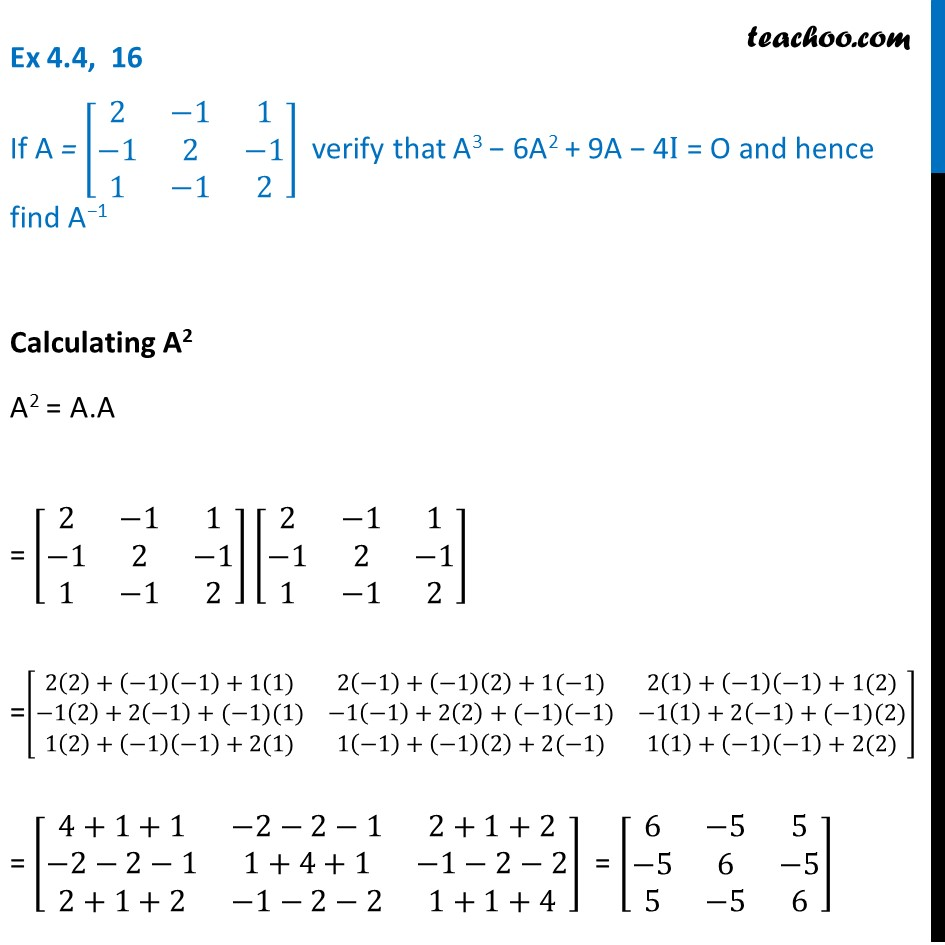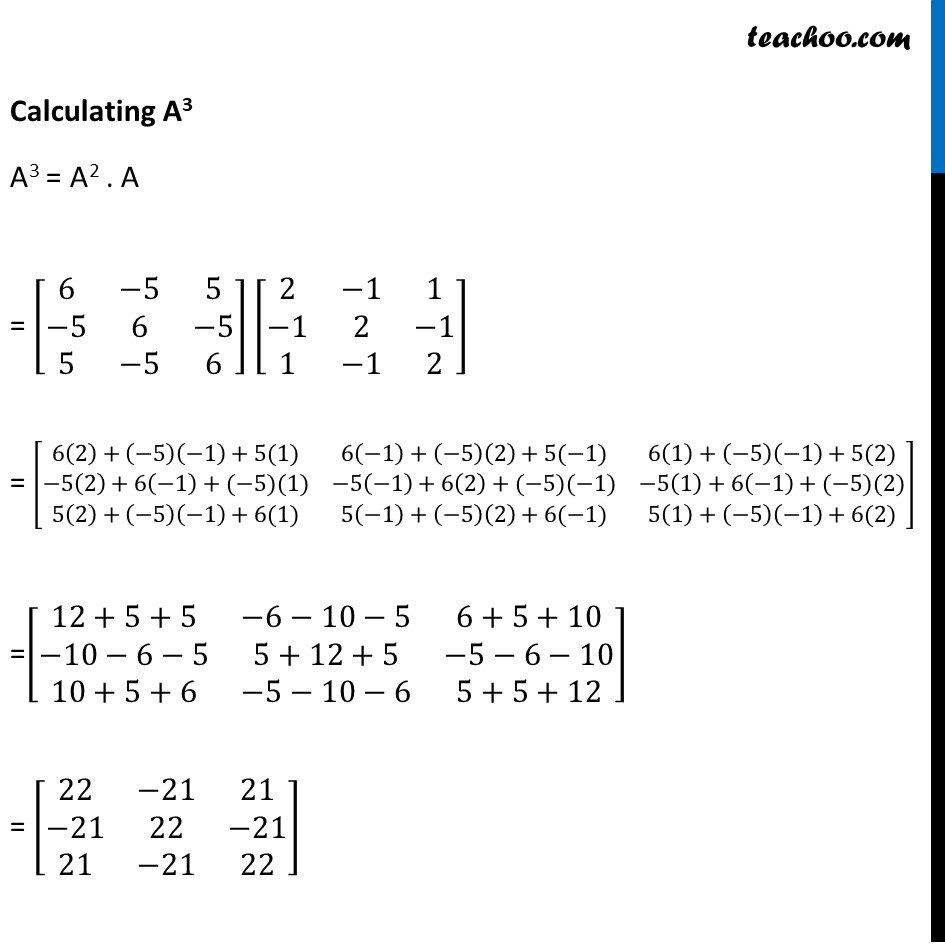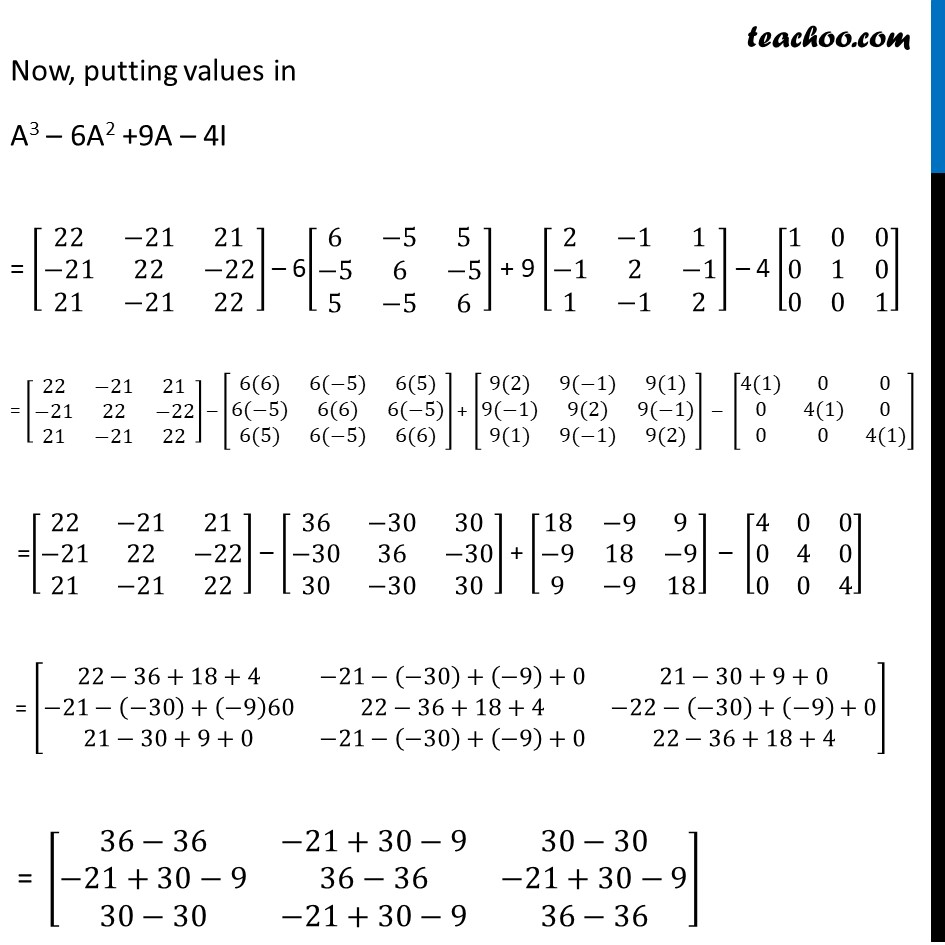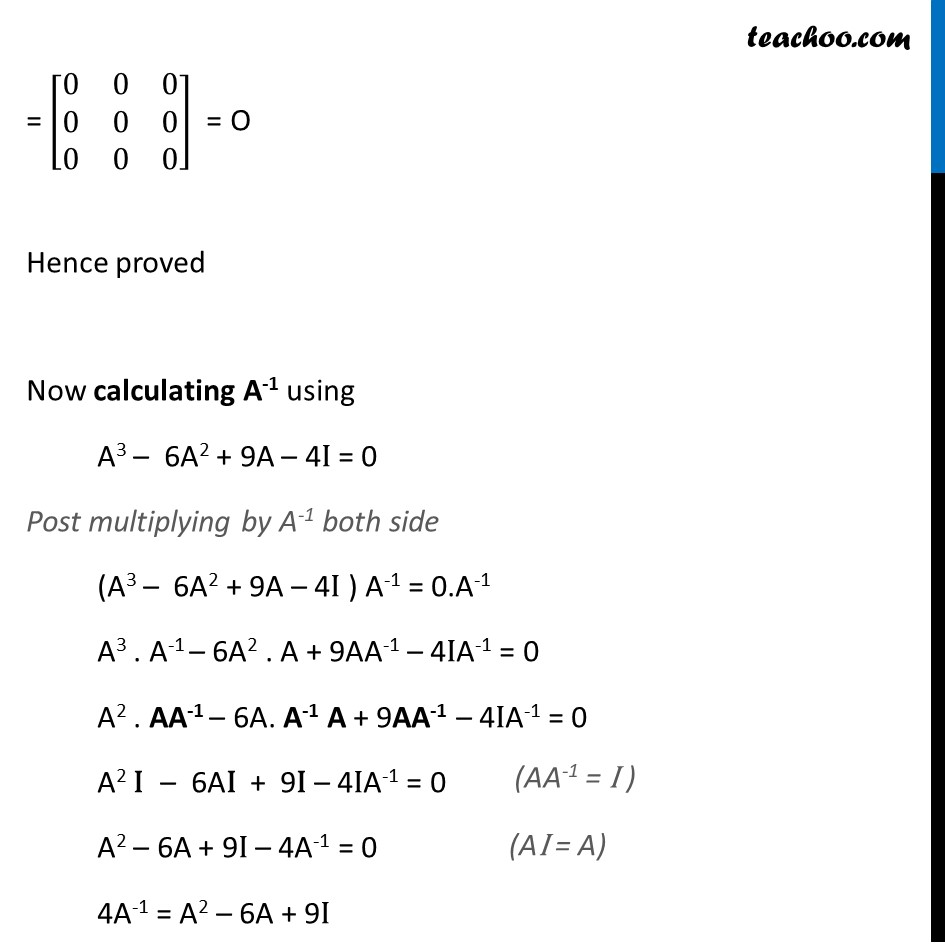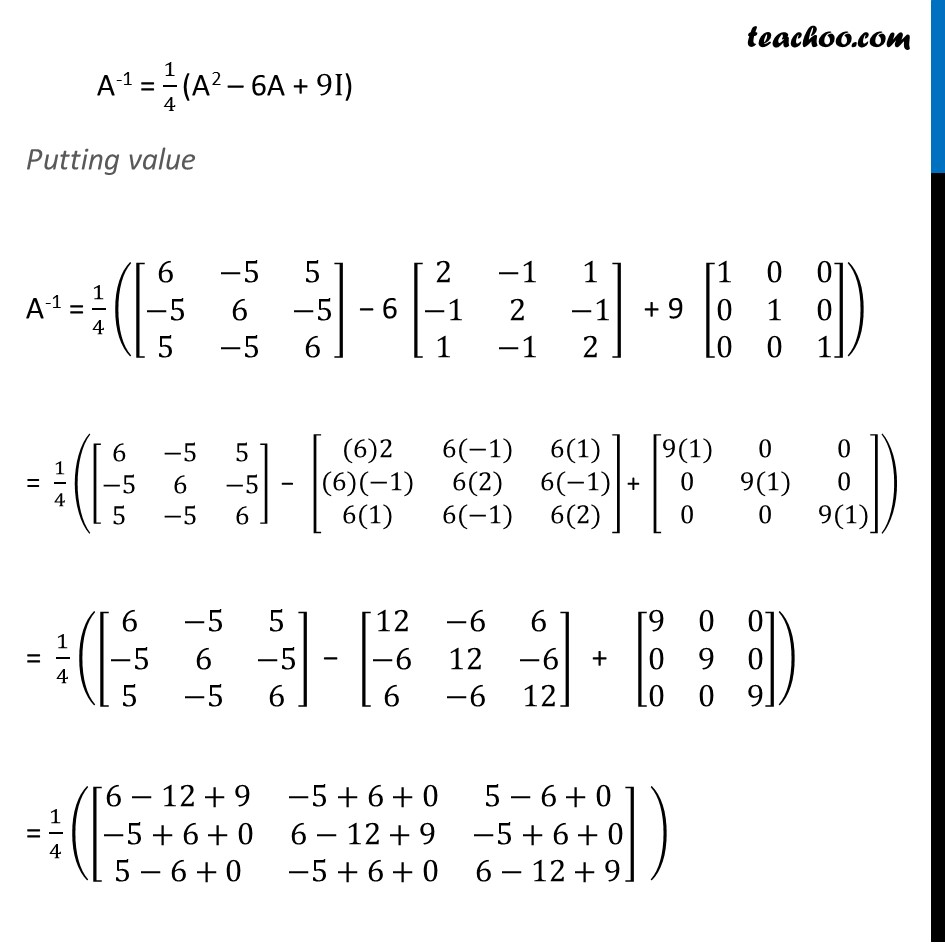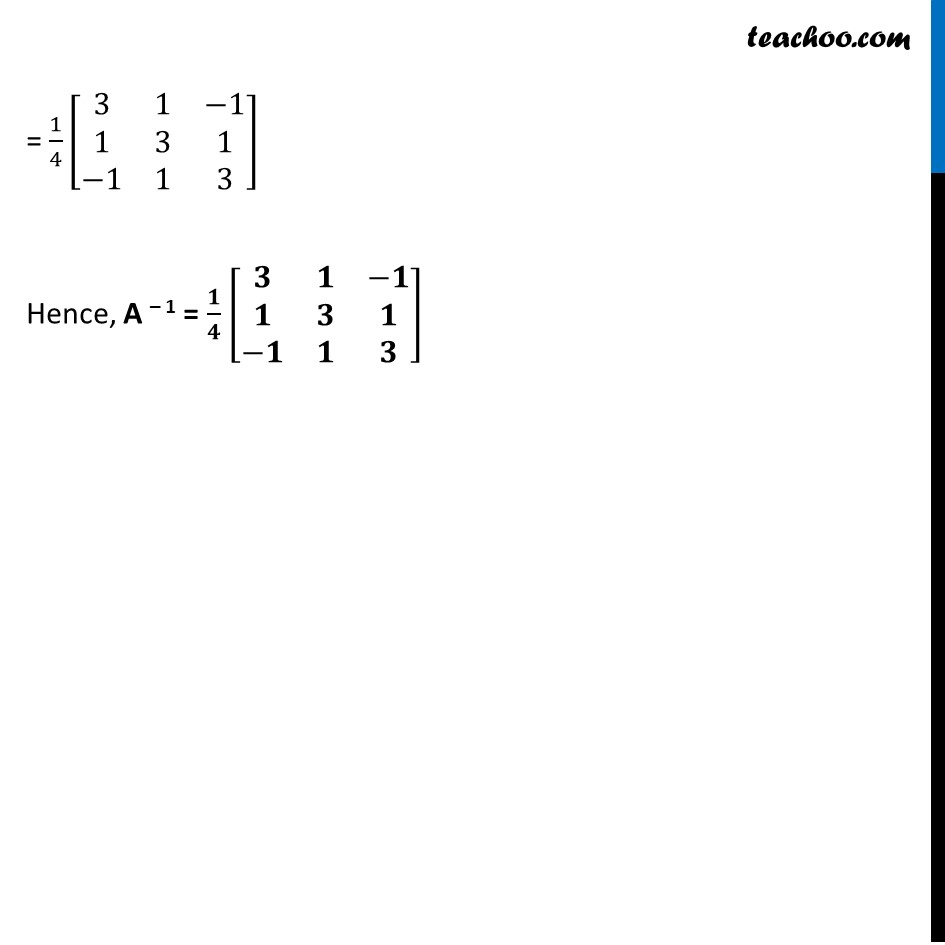Learn in your speed, with individual attention - Teachoo Maths 1-on-1 Class

### Transcript

Ex 4.4, 16 If A = [■8(2&−1&1@−1&2&−1@1&−1&2)] verify that A3 − 6A2 + 9A − 4I = O and hence find A−1 Calculating A2 A2 = A.A = [■8(2&−1&1@−1&2&−1@1&−1&2)] [■8(2&−1&1@−1&2&−1@1&−1&2)] =[■8(2(2)+(−1)(−1)+1(1)&2(−1)+(−1)(2)+1(−1)&2(1)+(−1)(−1)+1(2)@−1(2)+2(−1)+(−1)(1)&−1(−1)+2(2)+(−1)(−1)&−1(1)+2(−1)+(−1)(2)@1(2)+(−1)(−1)+2(1)&1(−1)+(−1)(2)+2(−1)&1(1)+(−1)(−1)+2(2))] = [■8(4+1+1&−2−2−1&2+1+2@−2−2−1&1+4+1&−1−2−2@2+1+2&−1−2−2&1+1+4)] = [■8(6&−5&5@−5&6&−5@5&−5&6)] Calculating A3 A3 = A2 . A = [■8(6&−5&5@−5&6&−5@5&−5&6)] [■8(2&−1&1@−1&2&−1@1&−1&2)] = [■8(6(2)+(−5)(−1)+5(1)&6(−1)+(−5)(2)+5(−1)&6(1)+(−5)(−1)+5(2)@−5(2)+6(−1)+(−5)(1)&−5(−1)+6(2)+(−5)(−1)&−5(1)+6(−1)+(−5)(2)@5(2)+(−5)(−1)+6(1)&5(−1)+(−5)(2)+6(−1)&5(1)+(−5)(−1)+6(2))] =[■8(12+5+5&−6−10−5&6+5+10@−10−6−5&5+12+5&−5−6−10@10+5+6&−5−10−6&5+5+12)] = [■8(22&−21&21@−21&22&−21@21&−21&22)] Now, putting values in A3 – 6A2 +9A – 4I = [■8(22&−21&21@−21&22&−22@21&−21&22)] – 6[■8(6&−5&5@−5&6&−5@5&−5&6)] + 9 [■8(2&−1&1@−1&2&−1@1&−1&2)] – 4 [■8(1&0&0@0&1&0@0&0&1)] = [■8(22&−21&21@−21&22&−22@21&−21&22)] – [■8(6(6)&6(−5)&6(5)@6(−5)&6(6)&6(−5)@6(5)&6(−5)&6(6))] + [■8(9(2)&9(−1)&9(1)@9(−1)&9(2)&9(−1)@9(1)&9(−1)&9(2))] – [■8(4(1)&0&0@0&4(1)&0@0&0&4(1))] =[■8(22&−21&21@−21&22&−22@21&−21&22)] – [■8(36&−30&30@−30&36&−30@30&−30&30)] + [■8(18&−9&9@−9&18&−9@9&−9&18)] – [■8(4&0&0@0&4&0@0&0&4)] = [■8(22−36+18+4&−21−(−30)+(−9)+0&21−30+9+0@−21−(−30)+(−9)60&22−36+18+4&−22−(−30)+(−9)+0@21−30+9+0&−21−(−30)+(−9)+0&22−36+18+4)] = [■8(36−36&−21+30−9&30−30@−21+30−9&36−36&−21+30−9@30−30&−21+30−9&36−36)] = [■8(0&0&0@0&0&0@0&0&0)] = O Hence proved Now calculating A-1 using A3 – 6A2 + 9A – 4I = 0 Post multiplying by A-1 both side (A3 – 6A2 + 9A – 4I ) A-1 = 0.A-1 A3 . A-1 – 6A2 . A + 9AA-1 – 4IA-1 = 0 A2 . AA-1 – 6A. A-1 A + 9AA-1 – 4IA-1 = 0 A2 I – 6AI + 9I – 4IA-1 = 0 A2 – 6A + 9I – 4A-1 = 0 4A-1 = A2 – 6A + 9I A-1 = 1/4 (A2 – 6A + 9I) Putting value A-1 = 1/4 ([■8(6&−5&5@−5&6&−5@5&−5&6)]" − 6 " [■8(2&−1&1@−1&2&−1@1&−1&2)]" + 9 " [■8(1&0&0@0&1&0@0&0&1)]) = 1/4 ([■8(6&−5&5@−5&6&−5@5&−5&6)]" − " [■8((6)2&6(−1)&6(1)@(6)(−1)&6(2)&6(−1)@6(1)&6(−1)&6(2))]"+ " [■8(9(1)&0&0@0&9(1)&0@0&0&9(1))]) = 1/4 ([■8(6&−5&5@−5&6&−5@5&−5&6)]" − " [■8(12&−6&6@−6&12&−6@6&−6&12)]" + " [■8(9&0&0@0&9&0@0&0&9)]) = 1/4 ([■8(6−12+9&−5+6+0&5−6+0@−5+6+0&6−12+9&−5+6+0@5−6+0&−5+6+0&6−12+9)]" " ) = 1/4 [■8(3&1&−1@1&3&1@−1&1&3)] Hence, A – 1 = 𝟏/𝟒 [■8(𝟑&𝟏&−𝟏@𝟏&𝟑&𝟏@−𝟏&𝟏&𝟑)]